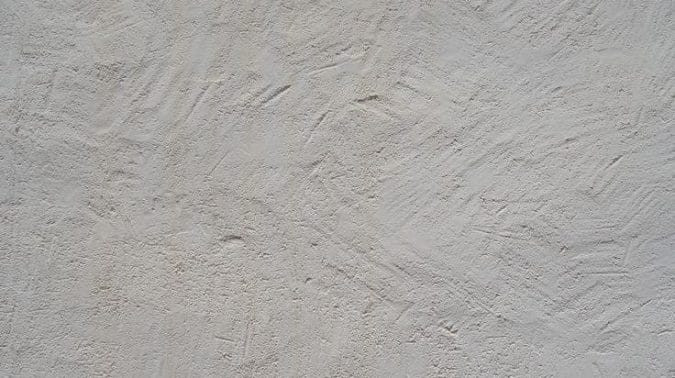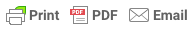Sorry, you do not have permission to ask a question, You must login to ask question. Become VIP Member

Do you need to remove the ads? Become VIP Member

Rate analysis of plastering with cement mortar requires the quantity estimation of materials cement mortar, i.e. quantity of cement, sand and water for various grades of mortar required. Grades of mortar used for plastering is generally same grade as used in the masonry work.

There are different grades of mortar that can be used for plastering of masonry structural members such as in CM 1:2, 1:4, 1:6, 1:8 etc. Cement Mortar in ratio 1:6 is generally used for plastering work.

For the calculation of quantity of cement and sand in mortar, volume of mortar required for plastering is calculated based on thickness of plastering surface and surface area of the structural member.Let us take an example of a wall to be plastered:

Length of wall = 2m

Width of wall = 1.5m

Thickness of plaster to be used = 20mm& Ad Free!

GET VIP MEMBERSHIP NOW!

Plaster to be carried out in two layers of 10mm each.

The quantity of cement mortar required will be: 2 x 1.5 x (20/1000) = 0.06 m3 of mortar.

The rate analysis of mortar need to be done for the calculated quantity required. Read here the rate analysis of cement mortar to know how to calculate quantity of cement and sand in mortar.

Once the quantity of cement and sand is calculated, the labor cost required for mixing of mortar is calculated. The coefficients of labor for plastering work are taken from the Rate Analysis by CPWD.

The cost of mixing of calculated quantity of mortar remains constant, but the cost of application of mortar varies with number of layers in which the mortar is applied. Thus, the cost of mason depends on area to be plastered and number of layers of plaster to be applied. The cost of other labors varies with the quantity of cement mortar to be mixed and number of layers of plasters to be applied.

The coefficients of mason and labors are as follows per m2 area per layer of plaster to be applied:

Mason – 0.07435 days

Labors – 0.0929 days

Bhishti – 0.0929 days (the one who carries materials and water for mixing)

These coefficients are multiplied with the given quantity of cement mortar required for plaster in m3 and number of layers or plasters.

The number of days of mason required = 0.07435 x No. of layers x surface area

= 0.07435 x 2 x (2 x1.5) = 0.44610 days

For Labors and Bhishti = 0.0929 x 2 x (2 x 1.5) = 0.5574 days for each.

The labor and bhishti days required for mixing of cement mortar is also calculated as given in rate analysis of cement mortar.

For example, labor required = 0.21 x 0.06 = 0.0126 days

Bhishti required = 0.0929 x 0.06 = 0.005574 days.

Thus total number of days for plastering in two-layers of plaster for 1.5mx2mx20mm plaster:

Mason = 0.44610 days

Labor = 0.5574 + 0.0126 = 0.57 days

Bhishti = 0.5574 + 0.005574 = 0.562974 days.

The daily wages of masons and labors are multiplied with number of days required by them to get the cost.

The excel calculation for rate analysis of plastering is shown below:

Rate Analysis of Civil Works -Elements and Requirements

Rate Analysis for Cement Mortar

Quantity of Cement & Sand Calculation in Mortar

Analysis of Rates for Civil Engineering Works

Rate Analysis for Brick Masonry

Special Plastering Materials for Different Purposes Used in Building Construction

Types of Plaster Finishes and External Rendering for Buildings